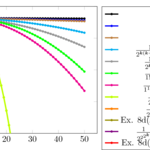# The strict superlinear order can be faster than the infinite order

## Abstract

The strict superlinear order (superlinear convergence) was usually regarded as having an intermediate speed between between linear and with order $$p>1$$.

In this paper we analyze the superlinear convergence (superlinear order/rate) of sequences and we show that actually there are four distinct classes of strict superlinear order: “weak”, “medium”, “strong” and “mixed”. The speed of the sequences from the first three classes is increasingly much faster, term-by-term big Oh, i.e.,
$|x^\ast-x_k|=\mathcal{O}(|y^\ast-y_k|^{\alpha}), \mbox{ as } k\rightarrow \infty, \forall \alpha>1 \mbox{ given},$whereas the speed of the “mixed” class cannot be assessed.

We prove that the speed of the sequences from the “medium” and “weak” classes is term-by-term slower than the speed of the sequences with high classical C-orders $$p>1$$ (in the sense of big Oh above), while an example shows that certain sequences from the “mixed” class may be term-by-term faster than some sequences with infinite C-order.

We also show that for a given sequence with strict superlinear convergence, one can evaluate numerically to which class it belongs.

Some recent results of Rodomanov and Nesterov (Math. Program., 2022), resp. Ye et al. (Math. Program., 2023) show that certain classical quasi-Newton methods (DFP, BFGS and SR1) belong to the “weak” class.

## Authors

Emil Cătinaş
“Tiberiu Popoviciu” Institute of Numerical Analysis

## Keywords

convergent sequence, convergence order, convergence speed, superlinear order, Q-order, convergence rate, big Oh, quasi-Newton method, DFP, BFGS, SR1.

## Paper coordinates

E. Cătinaş, The strict superlinear order can be faster than the infinite order, Numer. Algor., (2023). https://doi.org/10.1007/s11075-023-01604-y

## PDF

##### Journal

Numerical Algorithms

Springer Nature

1017-1398

##### Online ISSN

1572-9265

 Ortega, J.M., Rheinboldt, W.C.: Iterative Solution of Nonlinear Equations in Several Variables. SIAM, PA (2000)
 Beyer, W.A., Ebanks, B.R., Qualls, C.R.: Convergence rates and convergence-order profiles for sequences. Acta Appl. Math. 20, 267–284 (1990)
 Brezinski, C., Vitesse de convergence d’une suite. Rev. Roumaine Math. Pures Appl. 30, 403–417 (1985)
 Potra, F.A., On Q-order and R-order of convergence. J. Optim. Theory Appl. 63(3), 415–431 (1989)
 Catinas, E., A survey on the high convergence orders and computational convergence orders of sequences. Appl. Math. Comput. 343, 1–20 (2019)
 Catinas, E., How many steps still left to x*? SIAM Rev. 63(3), 585–624 (2021)
 Brezinski, C., Accélération de la Convergence en Analyse Numérique. Springer-Verlag, Berlin (1977)
 Potra, F.A., Pták, V.,  Nondiscrete Induction and Iterative Processes. Pitman, Boston, Massachusetts (1984)
 Tapia, R.A., Diagonalized multiplier methods and quasi-Newton methods for constrained optimization. J. Optim. Theory Appl. 22(2), 135–194 (1977)
 Polak, E., Optimization. Algorithms and Consistent Approximations. Springer-Verlag, New York (1997)
 Boggs, Paul T., Tolle, Jon W., Wang, Pyng, On the local convergence of Quasi-Newton methods for constrained optimization. SIAM Journal on Control and Optimization 20(2), 161–171 (1982)
 Tantau, T., The tikz and pgf packages. Manual for version 3.1.5b-34-gff02ccd1
 Bezanson, J., Edelman, A., Karpinski, S., Shah, V.B.: Julia: a fresh approach to numerical computing. SIAM Review 59, 65–98 (2017)
 Walker, H.F., An approach to continuation using Krylov subspace methods. In: Periaux, J. (ed.) Computational Science in the 21st Century, pp. 72–81. John Wiley and Sons, Ltd. (1997)
 Dennis, J.E., Jr., Moré, J.J., A characterization of superlinear convergence and its application to quasiNewton methods. Math. Comp. 28(126), 549–560 (1974)
 Rodomanov, A., Nesterov, Y., Rates of superlinear convergence for classical quasi-Newton methods. Math. Program. 194(1–2, Ser. A), 159–190 (2022)
 Ye, H., Lin, D., Chang, X., Zhang, Z., Towards explicit superlinear convergence rate for SR1. Math. Program. 199(1–2, Ser. A), 1273–1303 (2023)
 Catinas, E., Characterizing the classical convergence orders. Manuscript, submitted (2021)
 Catinas, E., Characterizing the classical linear convergence order Manuscript. (2021)
 Catinas, E., Stan, A., Measuring the measures. (2021). (manuscript)
 Stan, A., Catinas, E., Simpler proofs for the Q-order. Manuscript (2021)Linear convergence orders, weak superlinear convergence orders, medium superlinear convergence orders, strong superlinear convergence orders, mixed superlinear convergence orders, quadratic superlinear convergence orders and infinite superlinear convergence ordersVerifying numerically the superlinear convergence order / the superlinear order / the superlinear rate.